# Differential manifold

## Definition

### Definition in terms of atlases

A differential manifold or smooth manifold is the following data:

• A topological manifold$M$ (in particular,$M$ is Hausdorff and second-countable)
• An atlas of coordinate charts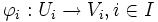$\varphi_i:U_i \to V_i, i \in I$ from$M$ to$\R^n$ (in other words an open cover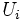$U_i$ of$M$ with homeomorphisms from each member$U_i$ of the open cover to open sets$V_i$ in$\R^n$)

satisfying the compatibility condition: the transition function between any two coordinate charts of the atlas is a diffeomomorphism of open subsets of$\R^n$. In symbols: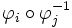$\varphi_i \circ \varphi_j^{-1}$

is a homeomorphism when restricted to the set: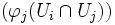$(\varphi_j(U_i \cap U_j))$

By diffeomorphism, we here mean a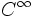$C^{\infty}$ map with a$C^{\infty}$ inverse.

However, we need to quotient out this data by the following equivalence:

Two atlases of coordinate charts on a topological space define the same differential manifold structure if taking their union still gives an atlas of coordinate charts. In other words, given any coordinate chart in one atlas and any coordinate chart in the other atlas, the transition function between them is a diffeomorphism.

### Definition in terms of sheaves

A differential manifold or smooth manifold is the following data:

• A topological manifold$M$
• A subsheaf of the sheaf of continuous functions from$M$ to$\R$, which plays the role of the sheaf of infinitely differentiable functions

Such that every point has a neighborhood with a homeomorphism to an open set in$\R^n$, such that the sheaf restriction to that open set, corresponds to the sheaf of infinitely differentiable functions on that open set.